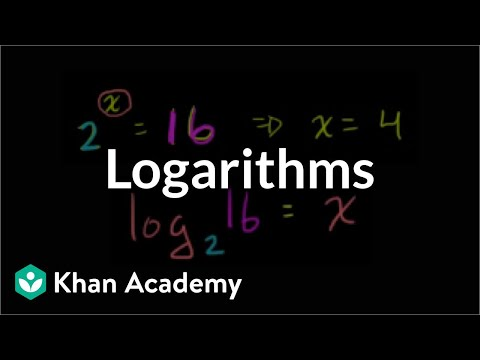### Algebra: Logarithms

Free Closed [?]Logarithms. Ex: Converting an exponential to logarithmic statement. Evaluating logarithms. Fancier logarithm expressions. Evaluating logarithms 2. Graphing Logarithmic Functions. Introduction to Logarithms. Introduction to logarithm properties. Introduction to logarithm properties (part 2). Logarithm of a Power. Sum of Logarithms with Same Base. Using Multiple Logarithm Properties to Simplify. Operations with logarithms. Change of Base Formula. Proof: log a + log b = log ab. Proof: A(log B) = log (B^A), log A - log B = log (A/B). Change of base formula proof. Logarithmic Equations. Solving Logarithmic Equations. Solving Logarithmic Equations. Logarithmic Scale. Richter Scale. Natural Logarithm with a Calculator. Calculator for Natural Logarithms. Graphing Natural Logarithm Function. Logarithms. Ex: Converting an exponential to logarithmic statement. Evaluating logarithms. Fancier logarithm expressions. Evaluating logarithms 2. Graphing Logarithmic Functions. Introduction to Logarithms. Introduction to logarithm properties. Introduction to logarithm properties (part 2). Logarithm of a Power. Sum of Logarithms with Same Base. Using Multiple Logarithm Properties to Simplify. Operations with logarithms. Change of Base Formula. Proof: log a + log b = log ab. Proof: A(log B) = log (B^A), log A - log B = log (A/B). Change of base formula proof. Logarithmic Equations. Solving Logarithmic Equations. Solving Logarithmic Equations. Logarithmic Scale. Richter Scale. Natural Logarithm with a Calculator. Calculator for Natural Logarithms. Graphing Natural Logarithm Function.

Categories:

# Reviews

-- no reviews yet -- make the first review

# Alternatives All alternatives to all Khan Academy courses

-- no alternatives found for the course --
If you know any alternatives, please let us know.

# Prerequisites

-- no prerequsites found for the course --
If you can suggest any prerequisite, please let us know.

# Paths

No Paths inclusing the course. You can build and share a path with this course included.

# Certification Exams

-- there are no exams to get certification after this course --
If your company does certification for those who completed this course then register your company as certification vendor and add your exams to the Exams Directory.

# Similar courses

Courses related to the course subject

### Advanced Algebraic Concepts and Applications in Mathematics

This course is ideal for people who want to gain a thorough understanding and knowledge of advanced topics in algebra. The advanc…

### Algebra

The foundations of Algebra from The Khan Academy.

### Algebra - Functions, Expressions and Equations

This free online course in algebra from ALISON will guide you through among other things expressions, systems of equations, functi…

### Algebra I

This undergraduate level Algebra I course covers groups, vector spaces, linear transformations, symmetry groups, bilinear forms, a…

### Algebra II

This undergraduate level course follows Algebra I. Topics include group representations, rings, ideals, fields, polynomial rings,…

### Algebra in Mathematics

This free online course offers a comprehensive introduction to algebra and carefully explains the concepts of algebraic fractions.

### Algebra: Absolute value

Understanding absolute value and solving absolute value equations and inequalities. Absolute Value and Number Lines. Absolute Valu…

### Algebra: ck12.org Algebra 1 Examples

Select problems from ck12.org's Algebra 1 FlexBook (Open Source Textbook). This is a good playlist to review if you want to make…

### Algebra: Conic sections

Identifying and graphing circles, ellipses, parabolas, and hyperbolas. Introduction to Conic Sections. Recognizing conic sections.

### Algebra: Exponent expressions and equations

Solving exponential and radical expressions and equations. Using scientific notation and significant figures. Negative and Positiv…

Let us know when you did the course Algebra: Logarithms.

 Started on: Completed on: Your grade (if any):Comments:Add the course Algebra: Logarithms to My Personal Education Path.

 Start the course on: Duration of study: 1 Week 2 Weeks 3 Weeks 4 Weeks 5 Weeks 6 Weeks 7 Weeks 8 Weeks 10 Weeks 12 Weeks 16 Weeks Notes: